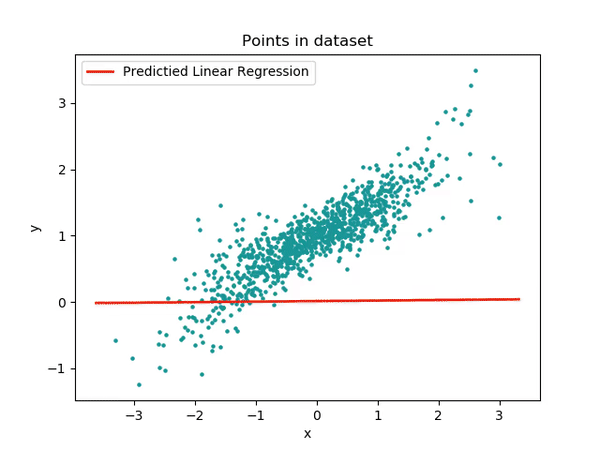# ML Club NIT Silchar# 7- Day Plan for a beginner in Machine Learning

### Day- 1

You have to start by learning some basics of a programming language. In Machine Learning we majorly use Python.If you want to learn Machine Learning with Python. You should know the below concepts:

• Variables

• Mathematical Operators

• Control Statements

• Data Structures (List, Set, Dict, etc.)

• Work with files

• Functions

• Object-Oriented Programming

Python Programming Beginner Tutorials - YouTube

Or you can watch this full video:

Python Tutorial - Python Full Course for Beginners - YouTube

Or you can do some online courses on Coursera, Udemy, etc.

### Day- 2

Let's introduce you to different libraries used in Machine Learning.On Day 2 you can just explore the most important libraries NumPy and Pandas.

NumPy is a Python library that allows you to work with multidimensional arrays, linear algebra, statistical operations and much more. NumPy means Numerical Python. Numpy provides us with a variety of features that simplify the handling of Python data.

Python NumPy Tutorial for Beginners - YouTube

### Day- 3:

Pandas is the top library you need to know before you get your hands on machine learning programming. Pandas is built on top of NumPy and well integrates with the scientific computing environment. It also supports time series-specific functionalities.When handling structured data, the first library you need is Pandas. You can achieve the following tasks and many more with the help of Pandas.

• Import the dataset into the workspace

• Identify the size and shape of the data

• Fetch rows and columns

• Handle missing values

• Analyze NaN values

• Groupby operations

• Merge and join datasets

• Data slicing (fetching a particular portion of the dataset), indexing

It ticks every checkbox of a data scientist’s needs – data cleaning, data analysis, and data organization. You can take away a perfect dataset from here for your data plotting and data modeling needs.

The following playlist will help you in learning the basics of Pandas:

Data analysis in Python with pandas - YouTube

### Day- 4:

Now before applying different machine learning algorithms we should know the math behind them.

You should know the following concepts:

• Categorical & Numerical Data

• Mean, Mode and Median

• Standard Deviation and Variance

• Co-Variance

• Correlation

• Skewness

• Random Variables

• Distributions

• Classic Probability

• Conditional ProbabilityMost importantly statistics. The following playlist covers the basics of statistics which will be needed (Videos 1- 10 ( Can watch all the videos of this playlist if you are interested) ):

### Day- 5:

Finally, you have to learn data visualization. Visualization takes a huge complex amount of data to represent charts or graphs for quick information to absorb and better understandability. It avoids hesitation on large data sets table to hold audience interest longer.The following playlist will cover all the basic visualization techniques to use:

### Day- 6:

There is one basic algorithm that you have to cover and that is Linear Regression.

Linear regression is a basic and commonly used type of predictive analysis. The simplest form of the regression equation with one dependent and one independent variable is defined by the formula y = c + b*x, where y = estimated dependent variable score, c = constant, b = regression coefficient, and x = score on the independent variable.

Three major uses for regression analysis are:

• Determining the strength of predictors

• Forecasting an effect

• Trend forecastingThe following playlist covers the basics of Linear Regression Full Lecture 4 (After completing this 7-day plan, I recommend you to watch this full playlist:

Machine Learning — Andrew Ng, Stanford University [FULL COURSE] - YouTube